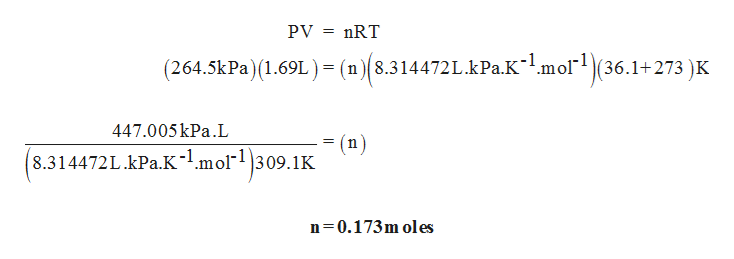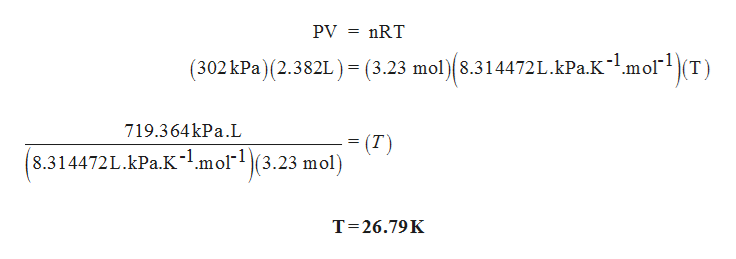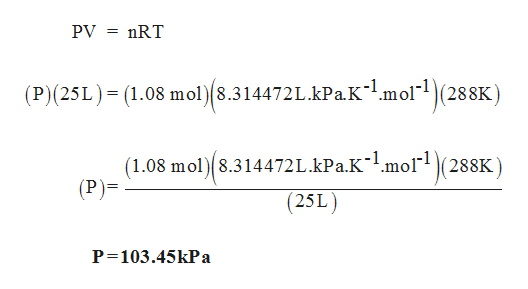For each set of values, calculate the missing variable using the ideal gas equation. ????Set 1264.5 kPa1.69 L36.1 ∘C?1Set 2302 kPa2382 mL?23.23 molSet 3?30.0250 m3288 K1.08 molSet 4104 kPa?4303 K5.26 mol     ?1=  _________________ mol                        ?2= _________K?3=________________kPa?4=_________________L

Question

For each set of values, calculate the missing variable using the ideal gas equation.

 ? ? ? ? Set 1 264.5 kPa 1.69 L 36.1 ∘C ?1 Set 2 302 kPa 2382 mL ?2 3.23 mol Set 3 ?3 0.0250 m3 288 K 1.08 mol Set 4 104 kPa ?4 303 K 5.26 mol

?1=  _________________ mol

?2= _________K

?3=________________kPa

?4=_________________L

Step 1

For Set 1: Moles is calculated as follows,help_outlineImage TranscriptioncloseРУ %3 nRT (264.5kPa) 1.69L) = (n)(8.314472 L.kPa.K-1.mol(36.1+273 )K 447.005kPa.L (n) 8.314472L.kPa.K-l.mol"|309.1K n 0.173m oles fullscreen
Step 2

For Set 2: Temperature is calculated as follows,help_outlineImage TranscriptionclosePV nRT (302kPa) (2.382L ) = (3.23 mol) 8.314472L.kPa.Kl.molT = 719.364kPa.L (T) 8.314472L.kPa.K1.mol(3.23 mol) T 26.79K fullscreen
Step 3

For Set 3: Pressure is cal...help_outlineImage TranscriptionclosePV nRT (P)(25L) (1.08 mol)8.314472L.kPa.Kmol(288K) (1.08 mol 8.314472L.kPa.K~mol(288K) (P)= (25L) P 103.45kPa fullscreen

Want to see the full answer?

See Solution

Want to see this answer and more?

Our solutions are written by experts, many with advanced degrees, and available 24/7

See Solution
Tagged in

General Chemistry